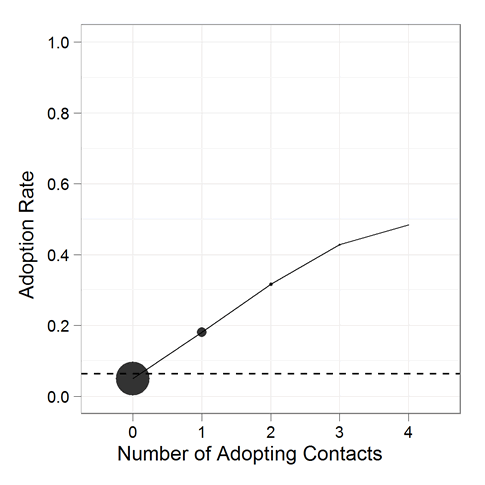# Posts Tagged ‘ plots ’

## introduction to R: learning by doing (part 2: plots)

July 10, 2012
ByLets go one with the second part of learning R by doing R (you will find the first part here. As we have used vectors, matrices and loops in the first part, we will concentrate on graphics in this one. but first we will need data to plot: Sometimes you will need several plots in

## Bare-bones intro to Plotting options in R

March 21, 2012
ByIf you’re using base::plot in R for the first time (for example if you do plot(pima) or plot(faithful) (use ??pima if you can’t find the dataset)) you may have looked at ?plot (2 page help file) or ?par (12 page help file) to figure out what’s go...

## Beeswarm Boxplot (and plotting it with R)

March 10, 2011
By(The image above is called a “Beeswarm Boxplot” , the code for producing this image is provided at the end of this post) The above plot is implemented under different names in different softwares. This “Scatter Dot Beeswarm Box Violin – plot” (in the lack of an agreed upon term) is a one-dimensional scatter plot

## Once again, chart critics and graph gurus welcome

December 10, 2010
ByHOW TO DISPLAY A LINE PLOT WITH COUNT INFORMATION? In a previously-mentioned paper Sharad and your DSN editor are writing up, there is the above line plot with points. The area of each point shows the count of observations. It’s done in R with ggplot2 (hooray for Hadley). We generally like this type of plot,

## A different way to view probability densities

June 12, 2010
ByThe standard, textbook way to represent a density function looks like this: Perhaps you have seen this before? (Plot created in R, all source code from this post is included at the end). Not only will you find this plot in statistics books, you’ll also see it in medical texts, sociology, and even economics books.

## Changing the fonts in R plots

October 28, 2007
ByTweet I use R to do almost all of my statistical analysis and plotting. It has a very steep learning curve but once you get into it, it’s very powerful and you can customise almost anything. The only problem is that these changes are not always obvious or easy to do. Case in point: how to change...